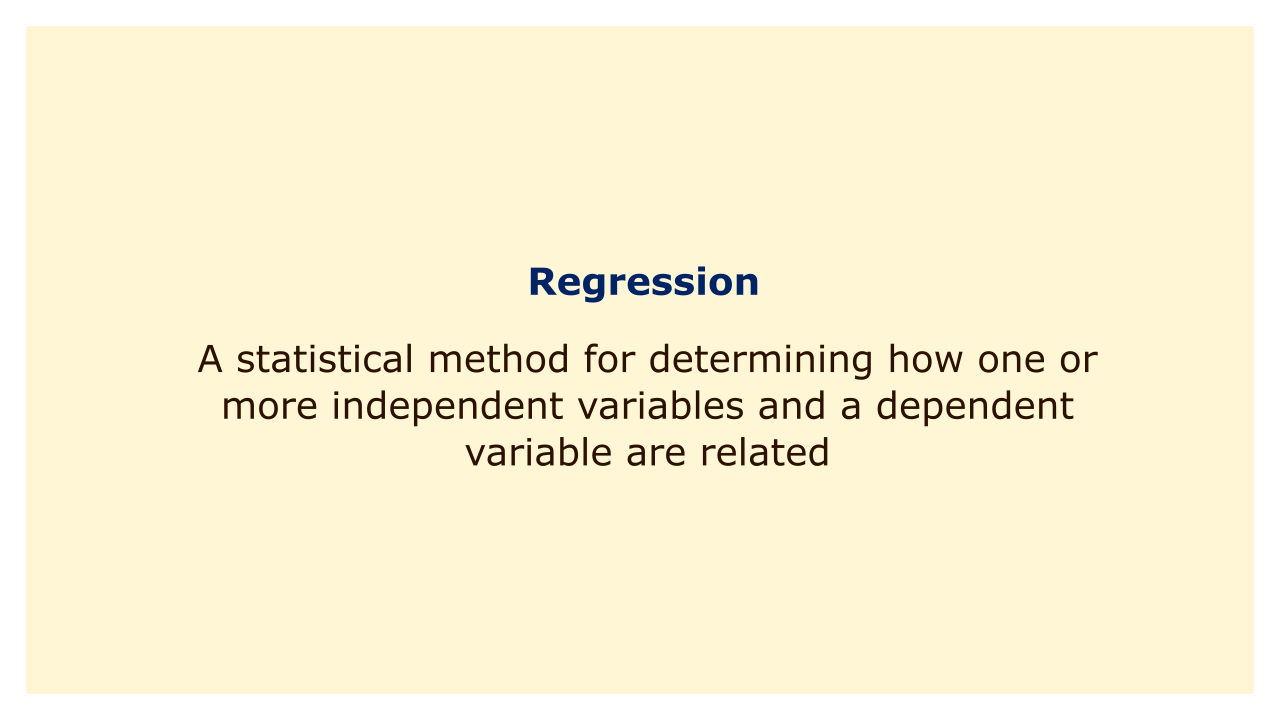# RegressionImage: Moneybestpal.com

### Regression is a statistical method for determining how one or more independent variables and a dependent variable are related. Creating a mathematical equation that may be utilized to forecast the value of the dependent variable based on the values of the independent variables is the aim of regression analysis.

Regression analysis comes in two primary flavors: simple regression and multiple regression. One independent variable is used in simple regression, but two or more independent variables are used in multiple regression.

Regression analysis is frequently used in finance to calculate the correlation between a financial variable and one or more economic variables. Regression analysis could be used, for instance, to ascertain the connection between a company's stock price and the overall performance of the stock market.

Ordinary Least Squares (OLS) regression is the most often used approach to regression analysis. By minimizing the sum of the squared differences between the predicted values and the actual values of the dependent variable, this approach determines the line of best fit.

Regression analysis can be used to forecast future values of the dependent variable, test hypotheses regarding the relationships between variables, and determine the direction and strength of those relationships. It has many uses in economics and finance, including portfolio management, risk management, and asset pricing.
Tags